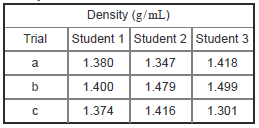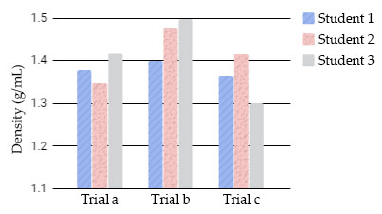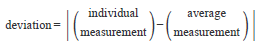# Problem: Part A. Rank the data sets in decreasing order of precision given the equation before the part.a. Student 1b. Student 2c. Student 3 In a laboratory experiment, the density of a concentrated sugar solution was determined by measuring the volume of the solution and corresponding mass. Three students each made a set of measurements using a different balance and used the results to calculate density. Their results are summarized in the table and bar graph.Precision describes the reproducibility of a measurement. To evaluate the precision of a set of measurements, start by finding the deviation of each individual measurement in the set from the average of all the measurements in the set:Note that deviation is always positive because the vertical lines in the formula represent absolute value. The average of all the deviations in the set is called the average deviation. The larger the average deviation is, the less precise is the data set.

###### FREE Expert Solution
81% (318 ratings)View Complete Written Solution
###### Problem Details

Part A. Rank the data sets in decreasing order of precision given the equation before the part.

a. Student 1

b. Student 2

c. Student 3

In a laboratory experiment, the density of a concentrated sugar solution was determined by measuring the volume of the solution and corresponding mass. Three students each made a set of measurements using a different balance and used the results to calculate density. Their results are summarized in the table and bar graph.Precision describes the reproducibility of a measurement. To evaluate the precision of a set of measurements, start by finding the deviation of each individual measurement in the set from the average of all the measurements in the set:Note that deviation is always positive because the vertical lines in the formula represent absolute value. The average of all the deviations in the set is called the average deviation. The larger the average deviation is, the less precise is the data set.